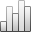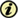Acta Stereologica

0351-580XMore Statisticssince 05 December 2013 :
View(s): 297 (1 ULiège)
Ervin E. Underwood

### Fractals in materials research

(Volume 13 (1994) — Number 2 - Proceedings of the sixth European congress for stereology - Part three - June 1994)
Article#### Abstract

The Richardson—Mandelbrot (R—M) fractal equation for lines (profiles and perimeters), and the Hack—Mandelbrot (H—M) fractal equation for perimeters and areas (lakes and islands) are examined closely in terms of the original derivations and assumptions. Of concern is the implicit assumption that theoretical results obtained for special geometrical figures also apply automatically to natural irregular curves. Discrepancies are found in the dimensionality of the equations, predictions of an infinite line length in the fractal plot, and lack of provisions to obtain a "true" line length. The fractal dimension D is not defined in physical terms. A serious limitation to the H—M expression is the requirement of constant island shape. The lack of commonality between the two fractal equations is most disturbing. Inconsistencies also arise between the theoretical predictions and the experimental findings. We observe nonlinear fractal plots and variable values of D, conflicting results for islands and lakes, and different results from vertical and horizontal sections. Solutions to some of these problems are proposed. The dimensionality shortcomings in the R—M and H—M equations are corrected, a linearization treatment is outlined for the reversed sigmoidal curves of materials fractal plots, an alternative equation is proposed that eliminates the restriction on island shapes, and suggestions are made to combine the two fractal equations of R—M and H—M into one.

Keywords : fractals, Hack—Mandelbrot, reverse sigmoidal fractal curves, Richardson—Mandelbrot, Tomkeieff